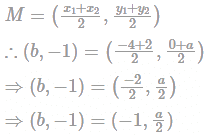Point Q(b,−1) is the midpoint of segment EF....

### Related TestPoint Q(b,−1) is the midpoint of segment EF. Co-ordinates of point E are (−4, a) and point F are (2,0). What is the value of a and b?Kunaal SatijaGiven,
Point Q(b,−1) is the midpoint of segment with endpoints E(−4, a) and F(2, 0).
As we know,
The midpoint of a segment with endpoints (x1, y1) and (x2, y2) is given by,On comparing, we get
b = −1, a = −2
Hence, the correct answer is a = -2, b = -1.View courses related to this question Explore CAT courses
 Explore CAT coursesView courses related to this question1 Crore+ students have signed up on EduRev. Have you?

• ### The Hi-Lo game is a four-player game played in six rounds. In every round, ... more(Scan QR code)